## Clean up TRACE Enhanced dataset

WRDS provides an excellent manual (link) and SAS code (link) for cleaning up the raw TRACE Enhanced bond transaction data, primarily based on the work done by Dick‐Nielsen, Jens, How to Clean Enhanced TRACE Data (December 3, 2014). Available at SSRN: https://ssrn.com/abstract=2337908. Dick‐Nielsen also provides his SAS code for the clean-up. Several papers refer to his cleaning steps.

Both WRDS and Dick-Nielsen’s codes remove cancellations, corrections, reversals, and double counting of agency trades. Dick-Nielsen’s code provides a few more options, e.g., remove commissioned trades.

## Stata command to perform Chow test

A Chow test is simply a test of whether the coefficients estimated over one group of the data are equal to the coefficients estimated over another.

I find two useful articles from Stata’s official website:

Suppose we do following regressions separately in two groups:

`regress y x1 x2 if group==1` and `regress y x1 x2 if group==2`

Then following commands will test the equality of coefficients on `x1` and `x2`:

`ge g2=(group==2)`
`regress y c.x1##i.g2 c.x2##i.g2`
`contrast g2 g2#c.x1 g2#c.x2, overall`

Stata’s official website gives an example of the output: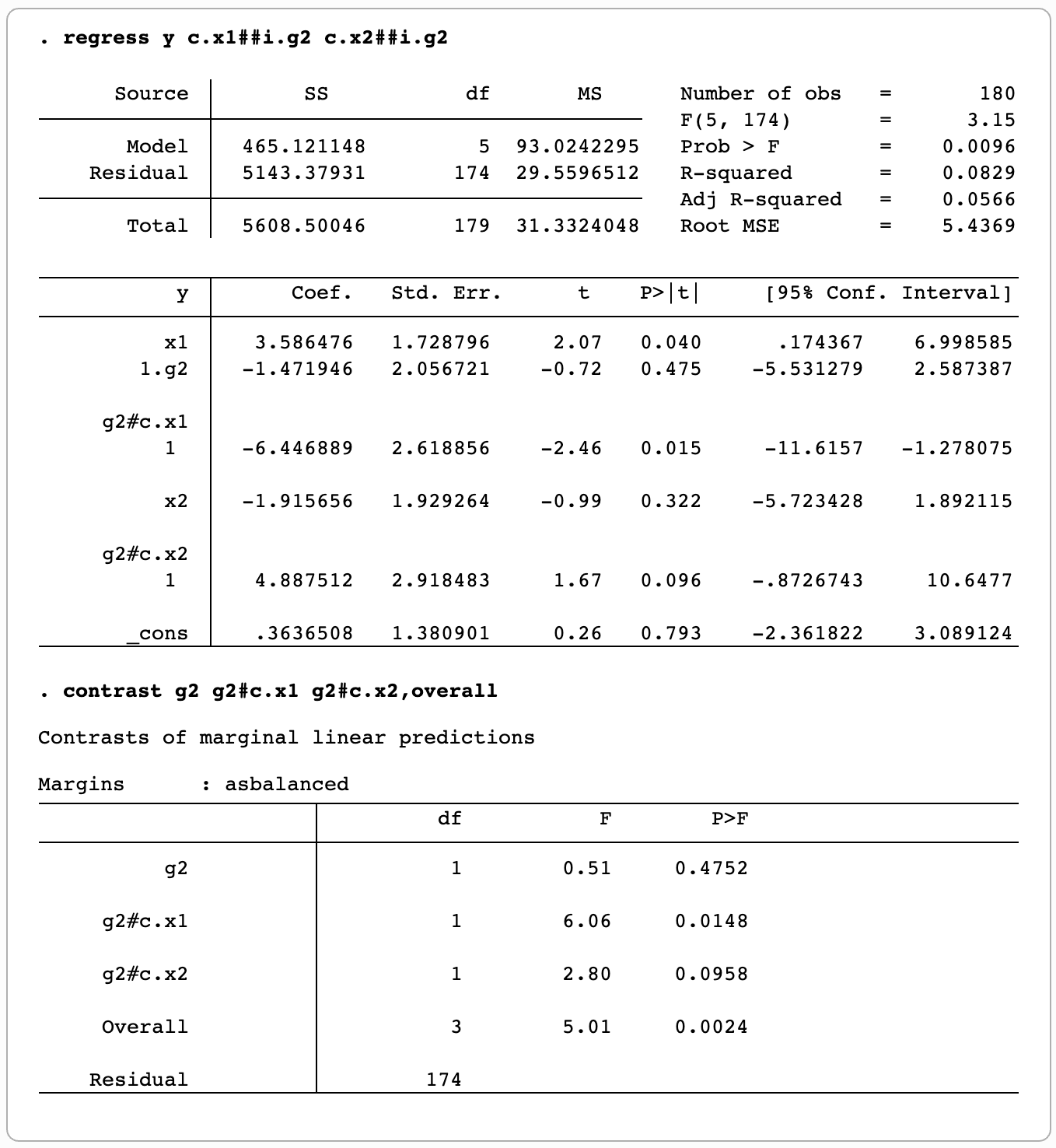In this example, to test the equality of coefficients on `x1` and `x2`, 6.06 and 2.80 are the F-stats that we are looking for.

Posted in Stata | 4 Comments

## SAS macro to count the number of analysts following a firm

This macro is used to count the number of analysts who followed a specific firm. Although this is a commonly used measure in literature, prior studies often give a vague description on what they do. The question is—what does “analysts following a firm” really mean?

First, it is only meaningful to count the number at a specified date.

Second, how to define “an analyst is actually following a firm”? I use the following definition: if an analyst issued any forecast (EPS or stock price or sales, anything) within a certain window (e.g., 180 days) before the specified date, then the analyst will be counted in. This definition ensures that the analyst is “actively” following the firm.

That is why my macro requires two arguments: DATE and WINDOW. This macro is used to answer such question—at a specified date, how many analysts are actively following Firm A, B, …?

## The art of regular expression

Regular expression is a powerful tool to do text search. It is the foundation of a lot of textual analysis research, though today’s textual analysis in computer science has gone far beyond text search. Regular expression operations are programming language independent. Any modern programming language supports regular expression operations well. So, if someone tells you that PERL is the best language to do text search (or textual analysis), that is plainly wrong.

Writing regular expression is work of art! You can find building blocks of regular expression here. I create this post to gather examples of regular expression that will solve certain text search questions. I will grow this post continuously.

Posted in Python | 1 Comment

## Stata commands to change variable names or values of string variables to all lowercase

Stata is a case-sensitive application. Sometimes this will cause a trouble. So, we may want to change variable names or values of variables to all lowercase before we start processing data. This post gives a fast way to do this.

Change variable names to all lowercase

We need to use the command `rename`. Instead of renaming variables one at a time, we can rename all variables in a single command (thanks Steve):

Change values of string variables to all lowercase

`ustrlower(string_variable)` or `strlower(string_variable)` will do the trick. Instead of applying `ustrlower` or `strlower` function to string variables one by one, we can benefit from lowercasing values of all string variables in a short loop. The following loop will first check the type of a variable. If it is a string variable, then change the value of the variable to all lowercase.

Posted in Stata | 2 Comments

## Common commands to deal with date in Stata

`egen compdatadate=eom(fiscalmonth fiscalyear)`
`format compdatadate %td`

To be continued …

## Stata command to order tabulation result with only top values shown

`tabulate varname` command is handy in Stata, but sometimes it returns a too long result, if `varname` contains too many unique values.

The third-party command, `groups`, will solve the problem by showing top values only. Please use `ssc install groups` to install `groups`. The usage of `group` is very similar to `tabulate`. Here are some examples:

## Empower “and” and “or” in IF statement in Stata

Stata is a little bit awkward when using `and` and `or` in `if` statement, compared to SAS. For example:

In SAS, we can write `if 2001 <= fyear <= 2010`. But in Stata, we usually write: `if fyear >= 2001 & fyear <= 2010`.

In fact, Stata provides a handy `inrange` function. The above `if` statement can be written as: `if inrange(fyear, 2001, 2010)`.

Similarly, Stata provides another `inlist` function. The syntax is `inlist(z, a, b, ...)`, which returns 1 if z = a or z = b … In `if` statement, it is equivalent to `if z = a | z = b | ...`

Posted in Stata | 1 Comment

## Display mean and median test results in Stata

Sometimes we may want to produce the following table to compare the mean and median of two groups: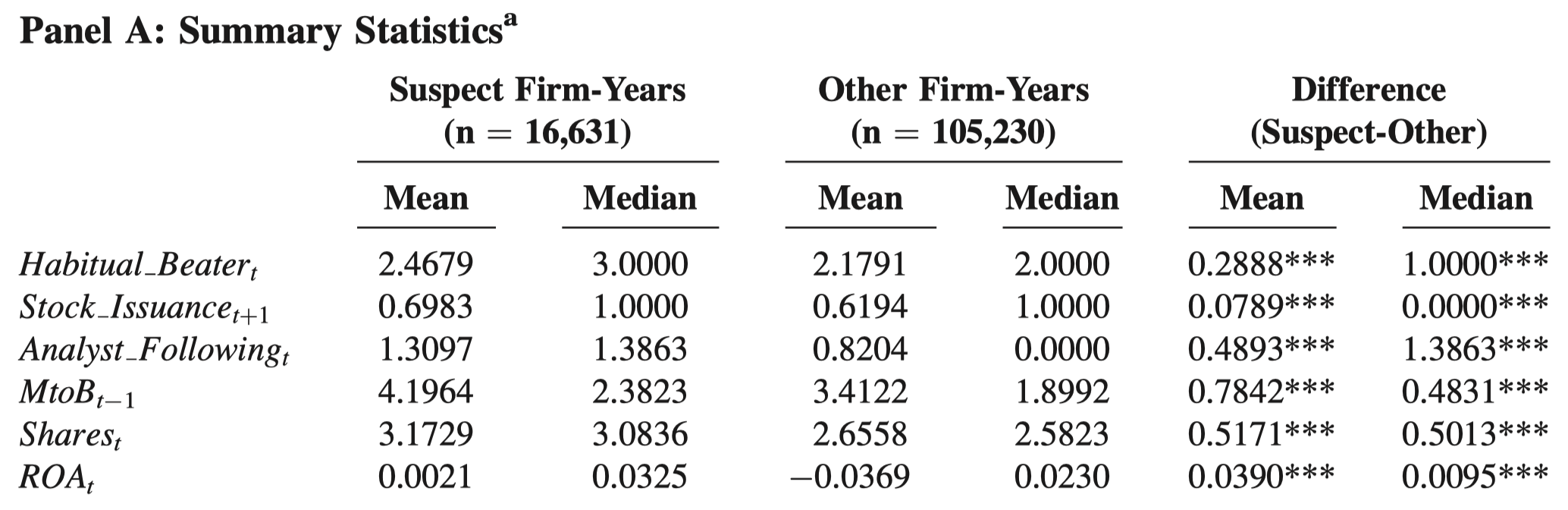First of all, please refer to this post to see Stata commands to test equality of mean and median.

However, it is time-consuming to glean numbers from the output of these Stata commands and place them in a table. It is even more struggling that you have to repeat the tedious process every time you update your sample.

I write Stata codes to streamline the process. The codes vary between unpaired (i.e., unmatched) data and paired data.

Unpaired data

The above example is unpaired data, i.e., suspect firm-years and other firm-years are not 1-to-1 or 1-to-m matched. One usage of unpaired data is the first step of Heckman’s two-step procedure, in which two groups of observations (i.e., the group that will be selected into the second step and the group that will not be selected into the second step) are stacked vertically in the dataset. The following codes are used for unpaired data. You only need to modify the first two lines to suit your data. The codes will generate a table in Stata’s output window like this: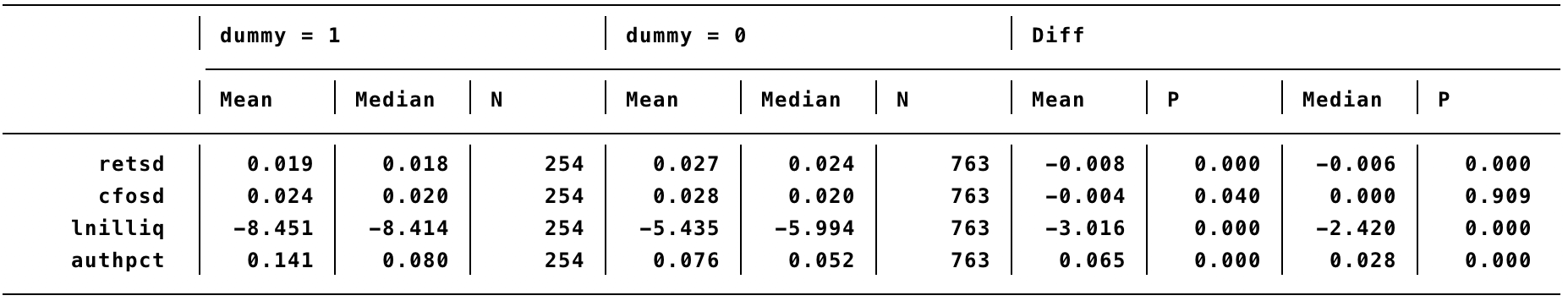You can then select the output and right-click “Copy as table” and paste in Excel for a quick edit. The codes use t-test for mean and Wilcoxon rank-sum test for median.

Paired data

A typical usage of paired data is to identify a matched control group for the treatment group. For example, identify a matched firm-year for an event firm-year based on a set of characteristics (same industry, same year, similar size and book-to-market), or identify a matched firm for every event firm based on the closest propensity score (i.e., propensity score matching).

The following table is an example that compares the mean and median of two matched groups—restating firms and non-restating group. Each restating firm is matched with a non-restating firm.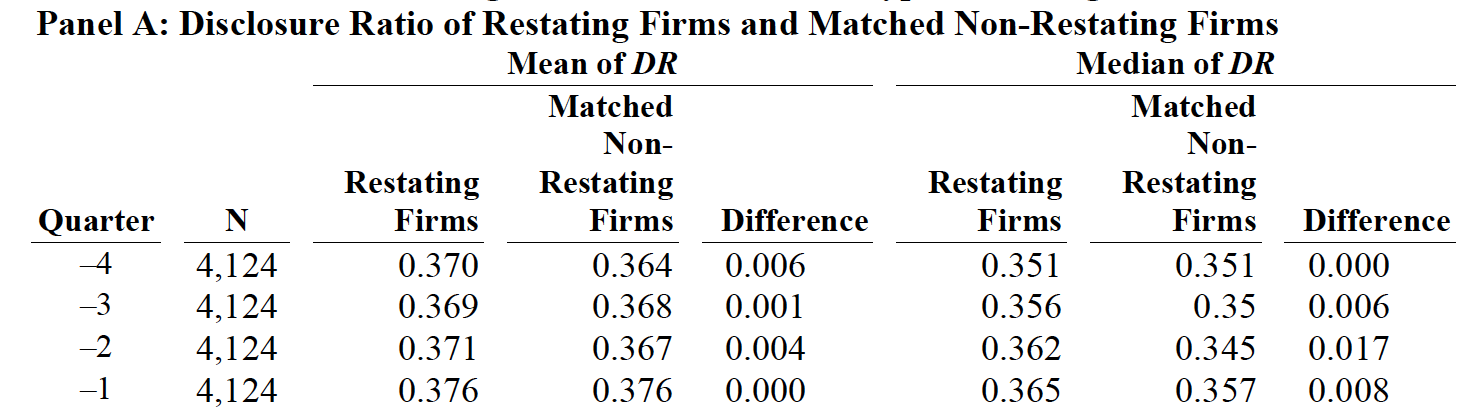Because of this matching relationship, every event firm and its control firm will be placed in the same row in the dataset. In other words, event firms and control firms are aligned horizontally. The following codes are used for paired data. You only need to modify the first two lines to suit your data. You must specify the same number of variables in the matched order in the first two lines. In other words, the first variable in the first line must be paired with the first variable in the second line, and so on. The codes will generate a table in Stata’s output window like this: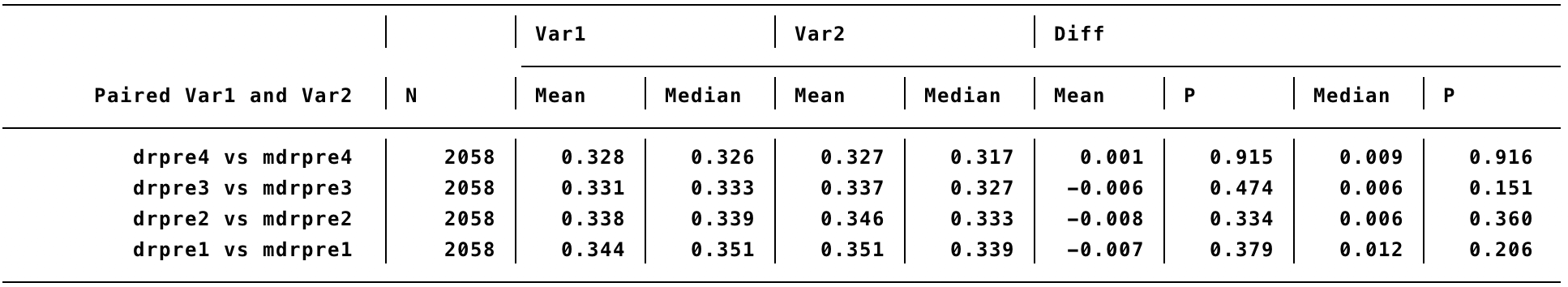The codes use paired t-test for mean and Wilcoxon rank-sign test for median.

Posted in Stata | 9 Comments

## Stata commands to do Heckman two steps

We often see Heckman’s two steps in accounting literature. But how to do it in Stata?

The two steps refer to the following two regressions:

Outcome equation: `y = X × b1 + u1`
Selection equation: `Dummy = Z × b2 + u2`

The selection equation must contain at least one variable that is not in the outcome equation.

The selection equation must be estimated using Probit. An intuitive way to do Heckman’s two steps is to estimate the selection equation first. Then include inverse mills ratio (IMR) derived from the selection equation in the outcome equation. In other words, run two regressions, one after the other.

Stata command for the selection equation:

`probit Dummy X` (using both observations that are selected into the sample and observations that are not selected into the sample, i.e., `Dummy` = 1 or `Dummy` = 0)

Note `vce` option (i.e., standard, robust or clustered standard errors, among others) will not change the resultant IMR.

Next, calculate IMR immediately:

`predict probitxb, xb`
`ge pdf = normalden(probitxb)`
`ge cdf = normal(probitxb)`
`ge imr = pdf/cdf`

Finally, include `imr` in the outcome equation:

`reg y X imr, vce(specified_vcetype)` (using observations that are selected into the sample only)

Note the first and the second regression use different numbers of observations.

However, this is not over. I find the first Probit regression sometimes causes missing IMR. For example, even if I have 100 observations with required `Dummy` and `X` data, I may only get IMR for 60 observations using this step-by-step method. I have not figured out why.

I then note that Stata in fact provides an all-in-one method to estimate both the selection equation and the outcome equation in one command `heckman`:

`heckman y X, select(Dummy = Z), twostep first mills(imr) vce(specfied_vcetype)`

I recommend using `twostep` option of the `heckman` command. This option will produce the same results with the step-by-step method. But this option may reduce the number of available `vce` types. In addition, the specified `vce` option only applies to the outcome equation and has no effect on the selection equation.

In this all-in-one method, we must pool together both observations that are selected into the sample and observations that are not selected into the sample, in which `Dummy` is 1 or 0 for all observations and `y` and `X` are missing for observations that are not selected into the sample. A benefit of this all-in-one method is that the weird missing-IMR issue will not appear.

I do have a closer look at missing IMR from the step-by-step method. They all have an extremely small value in the all-in-one method. I find that the step-by-step method has greater flexibility. Thus, if we want to use the step-by-step method but encounter the weird missing-IMR issue, it seems safe to just set missing IMR as zero.

Any comment is welcome.

Posted in Stata | 5 Comments